# 15  Measures of Spatial Autocorrelation

When analysing areal data, it has long been recognised that, if present, spatial autocorrelation changes how we may infer, relative to the default assumption of independent observations. In the presence of spatial autocorrelation, we can predict the values of observation $$i$$ from the values observed at $$j \in N_i$$, the set of its proximate neighbours. Early results entered into research practice gradually, for example the social sciences . These results were then collated and extended to yield a set of basic tools of analysis .

Cliff and Ord (1973) generalised and extended the expression of the spatial weights matrix representation as part of the framework for establishing the distribution theory for join-count, Moran’s $$I$$ and Geary’s $$C$$ statistics. This development of what have become known as global measures, returning a single value of autocorrelation for the total study area, has been supplemented by local measures returning values for each areal unit .

The measures offered by the spdep package have been written partly to provide implementations, but also to permit the comparative investigation of these measures and their implementation. For this reason, the implementations are written in R rather than compiled code, and they are generally slower but more flexible than implementations in the newly released rgeoda package .

## 15.1 Measures and process misspecification

It is not and has never been the case that Tobler’s first law of geography, “Everything is related to everything else, but near things are more related than distant things”, always holds absolutely. This is and has always been an oversimplification, disguising possible underlying entitation, support, and other misspecification problems. Are the units of observation appropriate for the scale of the underlying spatial process? Could the spatial patterning of the variable of interest for the chosen entitation be accounted for by another variable?

Tobler (1970) was published in the same special issue of Economic Geography as Olsson (1970), but Olsson does grasp the important point that spatial autocorrelation is not inherent in spatial phenomena, but often, is engendered by inappropriate entitation, by omitted variables and/or inappropriate functional form. The key quote from Olsson is on p. 228:

The existence of such autocorrelations makes it tempting to agree with Tobler (1970, 236 [the original refers to the pagination of a conference paper]) that ‘everything is related to everything else, but near things are more related than distant things.’ On the other hand, the fact that the autocorrelations seem to hide systematic specification errors suggests that the elevation of this statement to the status of ‘the first law of geography’ is at best premature. At worst, the statement may represent the spatial variant of the post hoc fallacy, which would mean that coincidence has been mistaken for a causal relation.

The status of the “first law” is very similar to the belief that John Snow induced from a map the cause of cholera as water-borne. It may be a good way of selling GIS, but it is inaccurate: Snow had a strong working hypothesis prior to visiting Soho, and the map was prepared after the Broad Street pump was disabled as documentation that his hypothesis held .

Measures of spatial autocorrelation unfortunately pick up other misspecifications in the way that we model data . For reference, Moran’s $$I$$ is given as :

$I = \frac{n \sum_{(2)} w_{ij} z_i z_j}{S_0 \sum_{i=1}^{n} z_i^2}$ where $$x_i, i=1, \ldots, n$$ are $$n$$ observations on the numeric variable of interest, $$z_i = x_i - \bar{x}$$, $$\bar{x} = \sum_{i=1}^{n} x_i / n$$, $$\sum_{(2)} = \stackrel{\sum_{i=1}^{n} \sum_{j=1}^{n}}{i \neq j}$$, $$w_{ij}$$ are the spatial weights, and $$S_0 = \sum_{(2)} w_{ij}$$. First we test a random variable using the Moran test, here under the normality assumption (argument randomisation=FALSE, default TRUE). Inference is made on the statistic $$Z(I) = \frac{I - E(I)}{\sqrt{\mathrm{Var}(I)}}$$, the z-value compared with the Normal distribution for $$E(I)$$ and $$\mathrm{Var}(I)$$ for the chosen assumptions; this x does not show spatial autocorrelation with these spatial weights:

library(spdep) |> suppressPackageStartupMessages()
library(parallel)
glance_htest <- function(ht) c(ht$estimate, "Std deviate" = unname(ht$statistic),
"Analytical randomisation" = unname(mtr$estimate)) # Permutation bootstrap Analytical randomisation # 0.000144 0.000140 Geary’s global $$C$$ is implemented in geary.test largely following the same argument structure as moran.test. The Getis-Ord $$G$$ test includes extra arguments to accommodate differences between implementations, as Bivand and Wong (2018) found multiple divergences from the original definitions, often to omit no-neighbour observations generated when using distance band neighbours. It is given by Getis and Ord (1992), on page 194. For $$G^*$$, the $$i \neq j$$ summation constraint is relaxed by including $$i$$ as a neighbour of itself (thereby also removing the no-neighbour problem, because all observations have at least one neighbour). Finally, the empirical Bayes Moran’s $$I$$ takes account of the denominator in assessing spatial autocorrelation in rates data . Until now, we have considered the proportion of valid votes cast in relation to the numbers entitled to vote by spatial entity, but using EBImoran.mc we can try to accommodate uncertainty in extreme rates in entities with small numbers entitled to vote. There is, however, little impact on the outcome in this case. Global measures of spatial autocorrelation using spatial weights objects based on graphs of neighbours are, as we have seen, rather blunt tools, which for interpretation depend critically on a reasoned mean model of the variable in question. If the mean model is just the intercept, the global measures will respond to all kinds of misspecification, not only spatial autocorrelation. The choice of entities for aggregation of data will typically be a key source of misspecification. ## 15.3 Local measures Building on insights from the weaknesses of global measures, local indicators of spatial association began to appear in the first half of the 1990s . In addition, the Moran plot was introduced, plotting the values of the variable of interest against their spatially lagged values, typically using row-standardised weights to make the axes more directly comparable . The moran.plot function also returns an influence measures object used to label observations exerting more than proportional influence on the slope of the line representing global Moran’s $$I$$. In Figure 15.1, we can see that there are many spatial entities exerting such influence. These pairs of observed and lagged observed values make up in aggregate the global measure, but can also be explored in detail. The quadrants of the Moran plot also show low-low pairs in the lower left quadrant, high-high in the upper right quadrant, and fewer low-high and high-low pairs in the upper left and lower right quadrants. In moran.plot, the quadrants are split on the means of the variable and its spatial lag; alternative splits are on zero for the centred variable and the spatial lag of the centred variable. Code I_turnout |> moran.plot(listw = lw_q_W, labels = pol_pres15$TERYT,
cex = 1, pch = ".", xlab = "I round turnout",
ylab = "lagged turnout") -> infl_W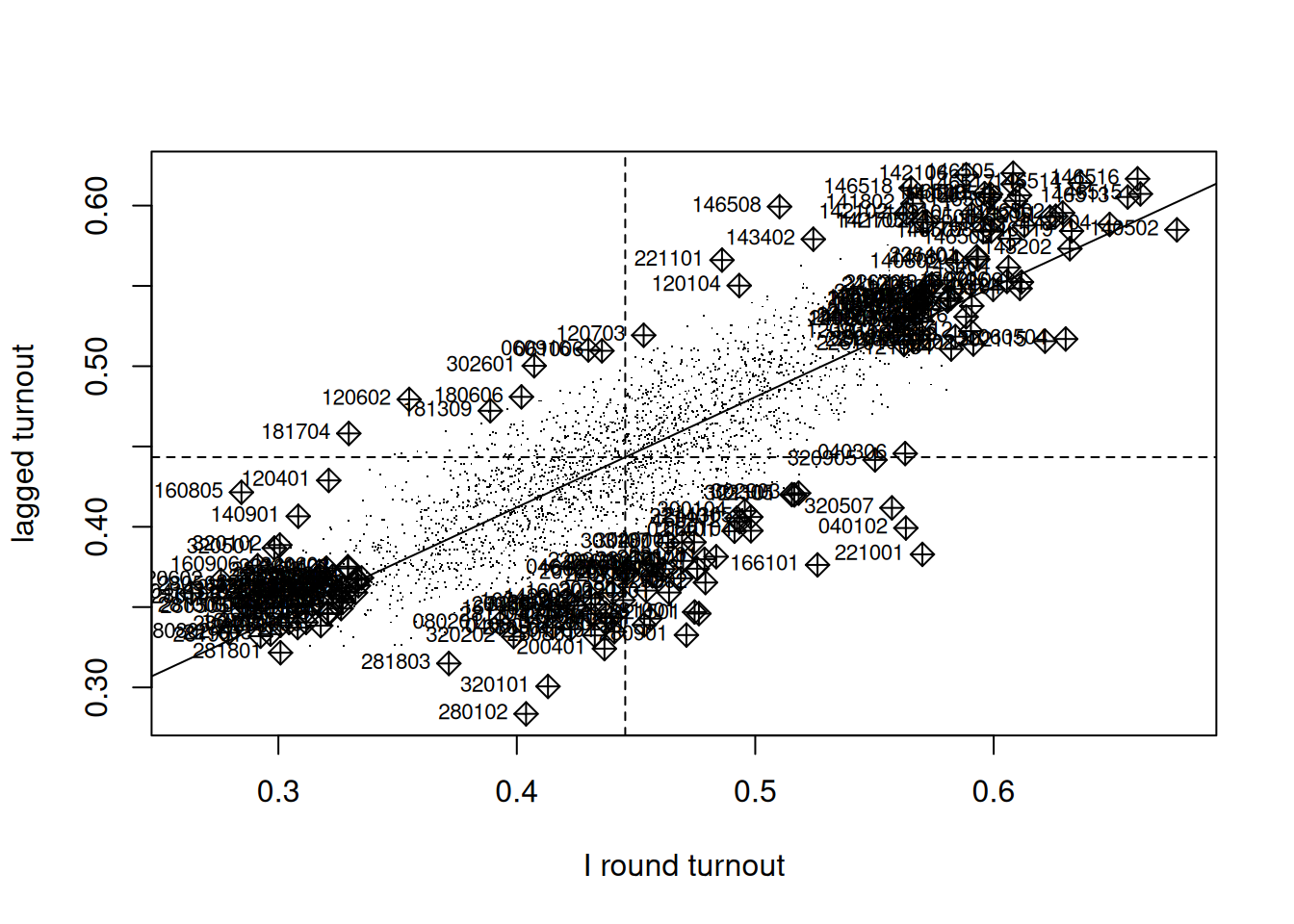Figure 15.1: Moran plot of I round turnout, row standardised weights

If we extract the hat value influence measure from the returned object, Figure 15.2 suggests that some edge entities exert more than proportional influence (perhaps because of row standardisation), as do entities in or near larger urban areas.

library(tmap)
pol_pres15$hat_value <- infl_W$hat
tm_shape(pol_pres15) + tm_fill("hat_value")

### Local Moran’s $$I_i$$

Bivand and Wong (2018) discuss issues impacting the use of local indicators, such as local Moran’s $$I_i$$ and local Getis-Ord $$G_i$$. Some issues affect the calculation of the local indicators, others inference from their values. Because $$n$$ statistics may be calculated from the same number of observations, there are multiple comparison problems that need to be addressed. Caldas de Castro and Singer (2006) conclude, based on a typical dataset and a simulation exercise, that the false discovery rate (FDR) adjustment of probability values will certainly give a better picture of interesting clusters than no adjustment. Following this up, Anselin (2019) explores the combination of FDR adjustments with the use of redefined “significance” cutoffs , for example $$0.01$$, $$0.005$$, and $$0.001$$ instead of $$0.1$$, $$0.05$$, and $$0.01$$; the use of the term interesting rather than significant is also preferred. This is discussed further in Bivand (2022a). As in the global case, misspecification remains a source of confusion, and, further, interpreting local spatial autocorrelation in the presence of global spatial autocorrelation is challenging .

args(localmoran)
#  function (x, listw, zero.policy = NULL, na.action = na.fail,
#    conditional = TRUE, alternative = "two.sided", mlvar = TRUE,
#    spChk = NULL, adjust.x = FALSE)

In an important clarification, Sauer et al. (2021) show that the comparison of standard deviates for local Moran’s $$I_i$$ based on analytical formulae and conditional permutation in Bivand and Wong (2018) was based on a misunderstanding. Sokal, Oden, and Thomson (1998) provide alternative analytical formulae for standard deviates of local Moran’s $$I_i$$ based either on total or conditional permutation, but the analytical formulae used in Bivand and Wong (2018), based on earlier practice, only use total permutation, and consequently do not match the simulation conditional permutations. Thanks to a timely pull request, localmoran now has a conditional= argument (default TRUE) using alternative formulae from the appendix of Sokal, Oden, and Thomson (1998). The mlvar= and adjust.x= arguments to localmoran are discussed in Bivand and Wong (2018), and permit matching with other implementations. Taking "two.sided" probability values (the default), we obtain:

I_turnout |>
localmoran(listw = lw_q_W) -> locm

The $$I_i$$ local indicators when summed and divided by the sum of the spatial weights equal global Moran’s $$I$$, showing the possible presence of positive and negative local spatial autocorrelation:

all.equal(sum(locm[,1])/Szero(lw_q_W),
unname(moran.test(I_turnout, lw_q_W)$estimate)) #  TRUE Using stats::p.adjust to adjust for multiple comparisons, we see that over 15% of the 2495 local measures have p-values < 0.005 if no adjustment is applied, but only 1.5% using Bonferroni adjustment to control the family-wise error rate, with two other choices shown: "fdr" is the Benjamini and Hochberg (1995) false discovery rate (almost 6%) and "BY" , another false discovery rate adjustment (about 2.5%): pva <- function(pv) cbind("none" = pv, "FDR" = p.adjust(pv, "fdr"), "BY" = p.adjust(pv, "BY"), "Bonferroni" = p.adjust(pv, "bonferroni")) locm |> subset(select = "Pr(z != E(Ii))", drop = TRUE) |> pva() -> pvsp f <- function(x) sum(x < 0.005) apply(pvsp, 2, f) # none FDR BY Bonferroni # 385 149 64 38 In the global measure case, bootstrap permutations may be used as an alternative to analytical methods for possible inference, where both the theoretical development of the analytical variance of the measure, and the permutation scheme, shuffle all of the observed values. In the local case, conditional permutation should be used, fixing the value at observation $$i$$ and randomly sampling from the remaining $$n-1$$ values to find randomised values at neighbours. Conditional permutation is provided as function localmoran_perm, which may use multiple compute nodes to sample in parallel if provided, and permits the setting of a seed for the random number generator across the compute nodes. The number of simulations nsim= also controls the precision of the ranked estimates of the probability value based on the rank of observed $$I_i$$ among the simulated values: library(parallel) invisible(spdep::set.coresOption(max(detectCores()-1L, 1L))) I_turnout |> localmoran_perm(listw = lw_q_W, nsim = 9999, iseed = 1) -> locm_p The outcome is that over 15% of observations have two sided p-values < 0.005 without multiple comparison adjustment, and about 1.5% with Bonferroni adjustment, when the p-values are calculated using the standard deviate of the permutation samples and the normal distribution. locm_p |> subset(select = "Pr(z != E(Ii))", drop = TRUE) |> pva() -> pvsp apply(pvsp, 2, f) # none FDR BY Bonferroni # 379 149 63 40 Since the variable under analysis may not be normally distributed, the p-values can also be calculated by finding the rank of the observed $$I_i$$ among the rank-based simulated values, and looking up the probability value from the uniform distribution taking the alternative= choice into account: locm_p |> subset(select = "Pr(z != E(Ii)) Sim", drop = TRUE) |> pva() -> pvsp apply(pvsp, 2, f) # none FDR BY Bonferroni # 394 125 0 0 Now the "BY" and Bonferroni counts of interesting locations are zero with 9999 samples, but may be recovered by increasing the sample count to 999999 if required; the FDR adjustment and interesting cutoff 0.005 yields about 5% locations. pol_pres15$locm_pv <- p.adjust(locm[, "Pr(z != E(Ii))"], "fdr")
pol_pres15$locm_std_pv <- p.adjust(locm_p[, "Pr(z != E(Ii))"], "fdr") pol_pres15$locm_p_pv <- p.adjust(locm_p[, "Pr(z != E(Ii)) Sim"],
"fdr")
Code
tm_shape(pol_pres15) +
tm_fill(c("locm_pv", "locm_std_pv", "locm_p_pv"),
breaks=c(0, 0.0005, 0.001, 0.005, 0.01,
0.05, 0.1, 0.2, 0.5, 0.75, 1),
title = "Pseudo p-values\nLocal Moran's I",
palette="-YlOrBr") +
tm_facets(free.scales = FALSE, ncol = 2) +
tm_layout(panel.labels = c("Analytical conditional",
"Permutation std. dev.",
"Permutation rank"))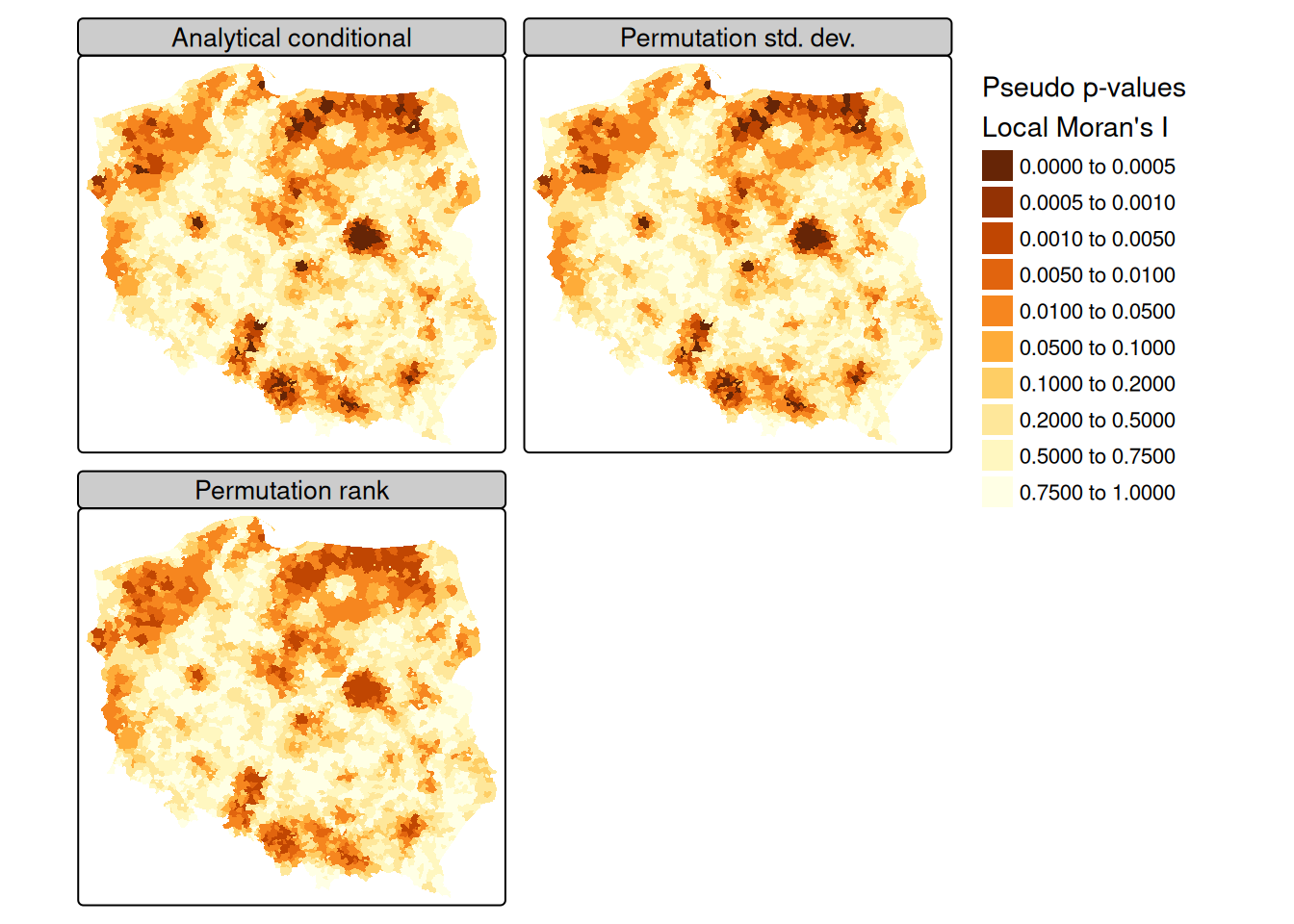Figure 15.3: Local Moran’s I FDR probability values: left upper panel: analytical conditional p-values; right upper panel: permutation standard deviate conditional p-values; left lower panel: permutation rank conditional p-values, first-round turnout, row-standardised neighbours

Proceeding using the FDR adjustment and an interesting location cutoff of $$0.005$$, we can see from Figure 15.3 that the adjusted probability values for the analytical conditional approach, the approach using the moments of the sampled values from permutation sampling, and the approach using the ranks of observed values among permutation samples all yield similar maps, as the distribution of the input variable is quite close to normal.

In presenting local Moran’s $$I$$, use is often made of “hotspot” maps. Because $$I_i$$ takes high values both for strong positive autocorrelation of low and high values of the input variable, it is hard to show where “clusters” of similar neighbours with low or high values of the input variable occur. The quadrants of the Moran plot are used, by creating a categorical quadrant variable interacting the input variable and its spatial lag split at their means. The quadrant categories are then set to NA if, for the chosen probability value and adjustment, $$I_i$$ would not be considered interesting. Here, for the FDR adjusted conditional analytical probability values (Figure 15.3, upper left panel), 53 observations belong to "Low-Low" cluster cores, and 96 to "High-High" cluster cores, similarly for the standard deviate-based permutation p-values (Figure 15.3, upper right panel), but the rank-based permutation p-values reduce the "High-High" count and increase the "Low-Low" count Figure 15.3 lower left panel:

quadr <- attr(locm, "quadr")$mean a <- table(addNA(quadr)) locm |> hotspot(Prname="Pr(z != E(Ii))", cutoff = 0.005, droplevels=FALSE) -> pol_pres15$hs_an_q
locm_p |> hotspot(Prname="Pr(z != E(Ii))", cutoff = 0.005,
droplevels=FALSE) -> pol_pres15$hs_ac_q locm_p |> hotspot(Prname="Pr(z != E(Ii)) Sim", cutoff = 0.005, droplevels = FALSE) -> pol_pres15$hs_cp_q
b <- table(addNA(pol_pres15$hs_an_q)) c <- table(addNA(pol_pres15$hs_ac_q))
d <- table(addNA(pol_pres15$hs_cp_q)) t(rbind("Moran plot quadrants" = a, "Analytical cond." = b, "Permutation std. cond." = c, "Permutation rank cond." = d)) # Moran plot quadrants Analytical cond. # Low-Low 1040 53 # High-Low 264 0 # Low-High 213 0 # High-High 978 96 # <NA> 0 2346 # Permutation std. cond. Permutation rank cond. # Low-Low 53 55 # High-Low 0 0 # Low-High 0 0 # High-High 96 70 # <NA> 2346 2370 pol_pres15$hs_an_q <- droplevels(pol_pres15$hs_an_q) pol_pres15$hs_ac_q <- droplevels(pol_pres15$hs_ac_q) pol_pres15$hs_cp_q <- droplevels(pol_pres15$hs_cp_q) Code tm_shape(pol_pres15) + tm_fill(c("hs_an_q", "hs_ac_q", "hs_cp_q"), colorNA = "grey95", textNA="Not \"interesting\"", title = "Turnout hotspot status \nLocal Moran's I", palette = RColorBrewer::brewer.pal(4, "Set3")[-c(2,3)]) + tm_facets(free.scales = FALSE, ncol = 2) + tm_layout(panel.labels = c("Analytical conditional", "Permutation std. cond.", "Permutation rank cond."))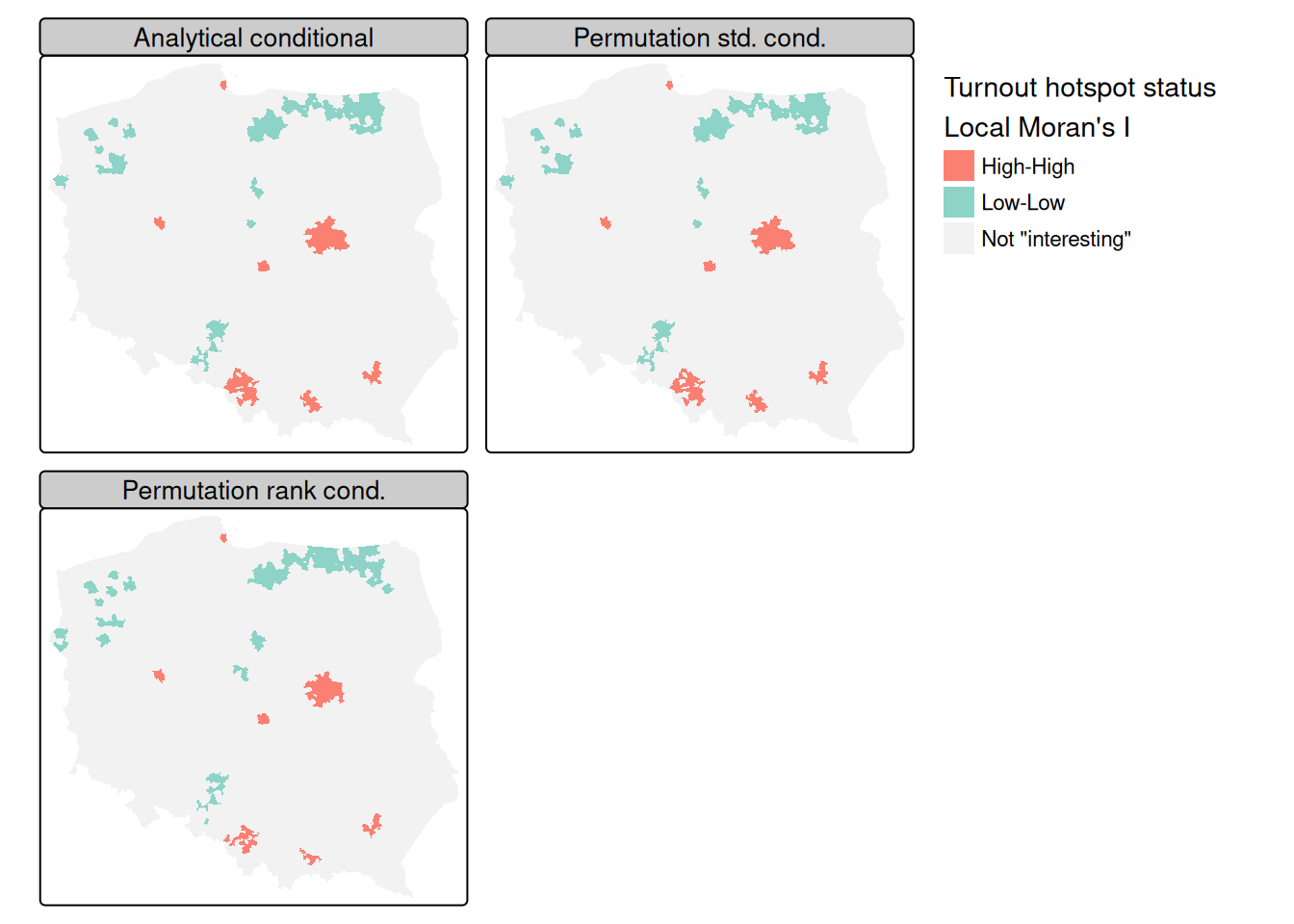Figure 15.4: Local Moran's I FDR hotspot cluster core maps $$\alpha = 0.005$$: left upper panel: analytical conditional p-values; right upper panel: permutation standard deviate conditional p-values; left lower panel: permutation rank conditional p-values, first-round turnout, row-standardised neighbours Figure 15.4 shows that there is very little difference between the FDR-adjusted interesting clusters with a choice of an $$\alpha=0.005$$ probability value cutoff for the three approaches of analytical conditional standard deviates, permutation-based standard deviates, and rank-based probability values; the "High-High" cluster cores are metropolitan areas. Tiefelsdorf (2002) argues that standard approaches to the calculation of the standard deviates of local Moran’s $$I_i$$ should be supplemented by numerical estimates, and shows that saddlepoint approximations are a computationally efficient way of achieving this goal. The localmoran.sad function takes a fitted linear model as its first argument, so we first fit a null (intercept only) model, but use case weights because the numbers entitled to vote vary greatly between observations: lm(I_turnout ~ 1) -> lm_null Saddlepoint approximation is as computationally intensive as conditional permutation, because, rather than computing a simple measure on many samples, a good deal of numerical calculation is needed for each local approximation: lm_null |> localmoran.sad(nb = nb_q, style = "W", alternative = "two.sided") |> summary() -> locm_sad_null The chief advantage of the saddlepoint approximation is that it takes a fitted linear model rather than simply a numerical variable, so the residuals are analysed. With an intercept-only model, the results are similar to local Moran’s $$I_i$$, but we can weight the observations, here by the count of those entitled to vote, which should down-weight small units of observation: lm(I_turnout ~ 1, weights = pol_pres15$I_entitled_to_vote) ->
lm_null_weights
lm_null_weights |>
localmoran.sad(nb = nb_q, style = "W",
alternative = "two.sided") |>
summary() -> locm_sad_null_weights

Next we add the categorical variable distinguishing between rural, urban and other types of observational unit:

lm(I_turnout ~ Types, weights=pol_pres15$I_entitled_to_vote) -> lm_types lm_types |> localmoran.sad(nb = nb_q, style = "W", alternative = "two.sided") |> summary() -> locm_sad_types locm_sad_null |> hotspot(Prname="Pr. (Sad)", cutoff=0.005) -> pol_pres15$locm_sad0
cutoff = 0.005) -> pol_pres15$locm_sad1 locm_sad_types |> hotspot(Prname="Pr. (Sad)", cutoff = 0.005) -> pol_pres15$locm_sad2
Code
tm_shape(pol_pres15) +
colorNA = "grey95", textNA = "Not \"interesting\"",
title = "Turnout hotspot status \nLocal Moran's I",
palette =RColorBrewer::brewer.pal(4, "Set3")[c(1, 4, 2)]) +
tm_facets(free.scales = FALSE, ncol = 2) +
tm_layout(panel.labels = c("Permutation rank",
"saddlepoint weighted types"))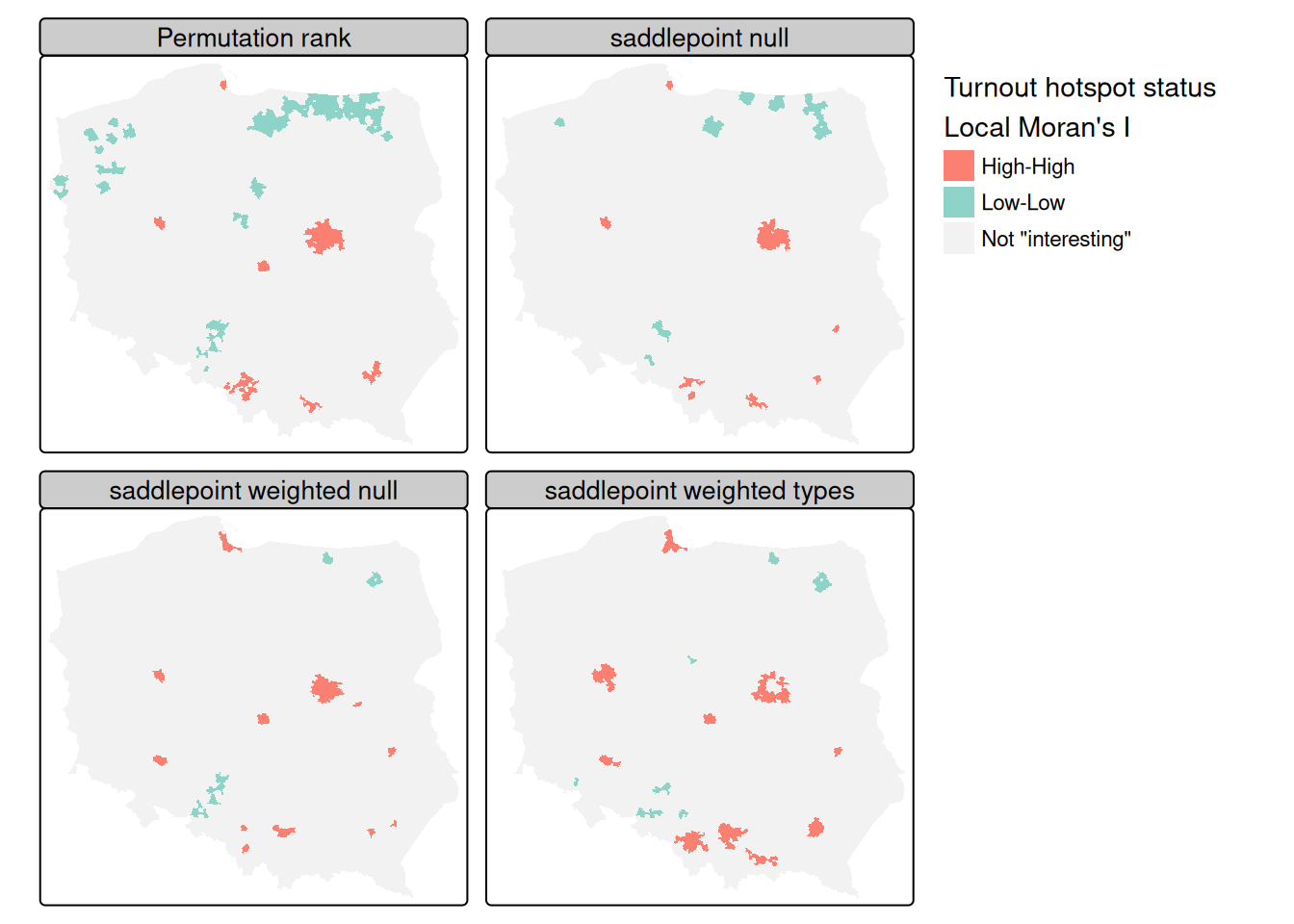Figure 15.5: Local Moran's I FDR hotspot cluster core maps, two-sided, interesting cutoff $$\alpha = 0.005$$: left upper panel: permutation rank conditional p-values; right upper panel: null (intercept only) model saddlepoint p-values; left lower panel: weighted null (intercept only) model saddlepoint p-values; right lower panel: weighted types model saddlepoint p-values, for first-round turnout, row-standardised neighbours
rbind(null = append(table(addNA(pol_pres15$locm_sad0)), c("Low-High" = 0), 1), weighted = append(table(addNA(pol_pres15$locm_sad1)),
c("Low-High" = 0), 1),
type_weighted = append(table(addNA(pol_pres15$locm_sad2)), c("Low-High" = 0), 1)) # Low-Low Low-High High-High <NA> # null 19 0 55 2421 # weighted 9 0 52 2434 # type_weighted 13 0 81 2401 Figure 15.5 includes the permutation rank cluster cores for comparison (upper left panel). Because saddlepoint approximation permits richer mean models to be used, and possibly because the approximation approach is inherently local, relating regression residual values at $$i$$ to those of its neighbours, the remaining three panels diverge somewhat. The intercept-only (null) model is fairly similar to standard local Moran’s $$I_i$$, but weighting by counts of eligible voters removes most of the "Low-Low" cluster cores. Adding the type categorical variable strengthens the urban "High-High" cluster cores but removes the Warsaw boroughs as interesting cluster cores. The central boroughs are surrounded by other boroughs, all with high turnout, not driven by autocorrelation but by being metropolitan boroughs. It is also possible to use saddlepoint approximation where the global spatial process has been incorporated, removing the conflation of global and local spatial autocorrelation in standard approaches. The same can also be accomplished using exact methods, but may require more tuning as numerical integration may fail, returning NaN rather than the exact estimate of the standard deviate : lm_types |> localmoran.exact(nb = nb_q, style = "W", alternative = "two.sided", useTP=TRUE, truncErr=1e-8) |> as.data.frame() -> locm_ex_types locm_ex_types |> hotspot(Prname = "Pr. (exact)", cutoff = 0.005) -> pol_pres15$locm_ex
Code
tm_shape(pol_pres15) +
textNA = "Not \"interesting\"",
title = "Turnout hotspot status \nLocal Moran's I",
palette = RColorBrewer::brewer.pal(4, "Set3")[c(1, 4, 2)]) +
tm_facets(free.scales = FALSE, ncol = 2) +
"Exact weighted types"))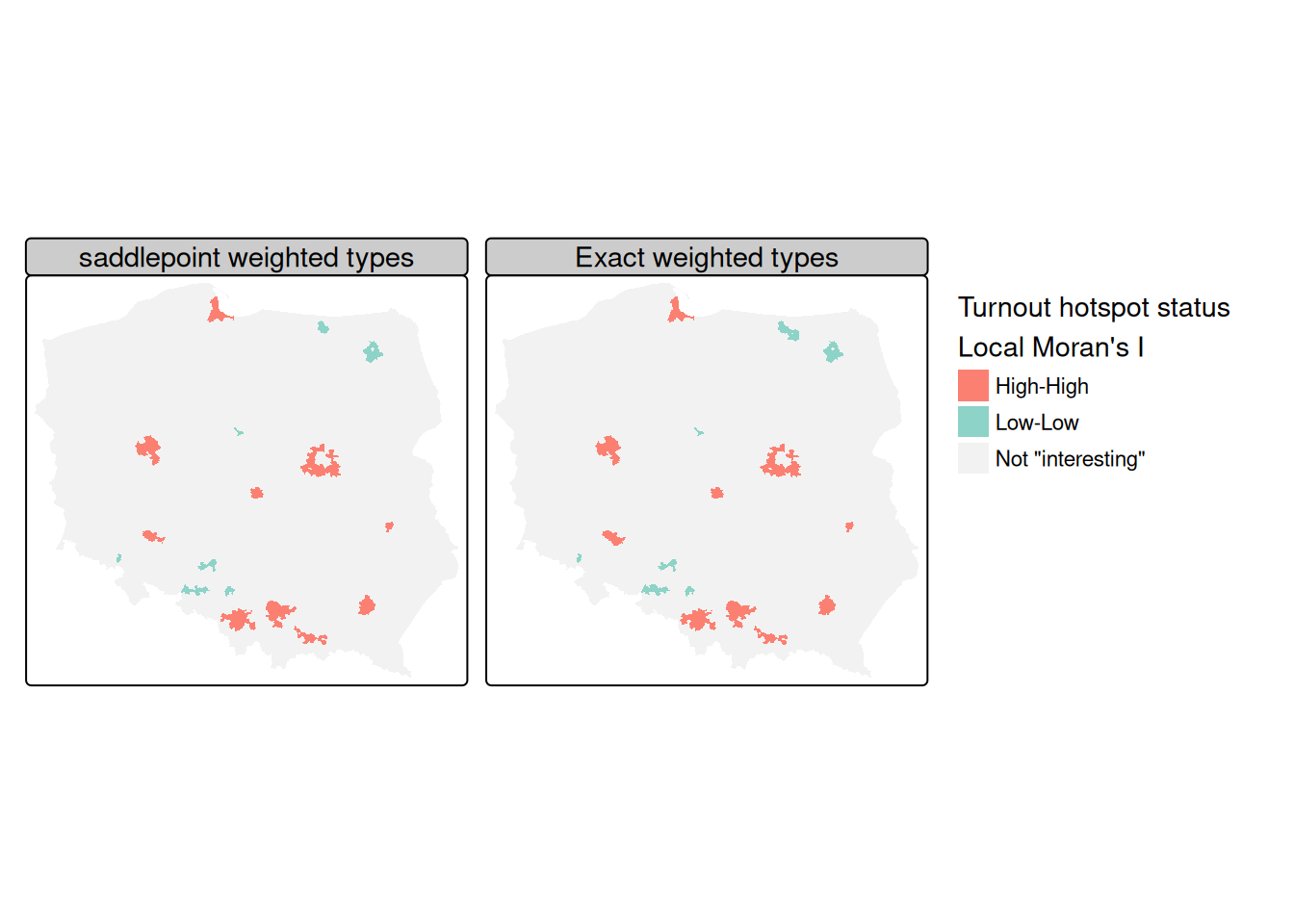Figure 15.6: Local Moran’s I FDR hotspot cluster core maps, two-sided, interesting cutoff $$\alpha = 0.005$$: left panel: weighted types model saddlepoint p-values; right panel: weighted types model exact p-values, for first-round turnout, row-standardised neighbours

As Figure 15.6 shows, the exact and saddlepoint approximation methods yield almost identical cluster classifications from the same regression residuals, multiple comparison adjustment method, and cutoff level, with the exact method returning four more interesting observations:

table(Saddlepoint = addNA(pol_pres15$locm_sad2), exact = addNA(pol_pres15$locm_ex))
#            exact
#   Low-Low        13         0    0
#   High-High       0        81    0
#   <NA>            2         2 2397

### Local Getis-Ord $$G_i$$

The local Getis-Ord $$G_i$$ measure is reported as a standard deviate, and, may also take the $$G^*_i$$ form where self-neighbours are inserted into the neighbour object using include.self. The observed and expected values of local $$G$$ with their analytical variances may also be returned if return_internals=TRUE.

I_turnout |>
localG(lw_q_W, return_internals = TRUE) -> locG

Permutation inference is also available for this measure:

I_turnout |>
localG_perm(lw_q_W, nsim = 9999, iseed = 1) -> locG_p

The correlation between the two-sided probability values for analytical and permutation-based standard deviates (first two columns and rows) and permutation rank-based probability values are very strong:

cor(cbind(localG=attr(locG, "internals")[, "Pr(z != E(Gi))"],
attr(locG_p, "internals")[, c("Pr(z != E(Gi))",
"Pr(z != E(Gi)) Sim")]))
#                    localG Pr(z != E(Gi)) Pr(z != E(Gi)) Sim
# localG                  1              1                  1
# Pr(z != E(Gi))          1              1                  1
# Pr(z != E(Gi)) Sim      1              1                  1

### Local Geary’s $$C_i$$

Anselin (2019) extends Anselin (1995) and has been recently added to spdep thanks to contributions by Josiah Parry (pull request https://github.com/r-spatial/spdep/pull/66). The conditional permutation framework used for $$I_i$$ and $$G_i$$ is also used for $$C_i$$:

I_turnout |>
localC_perm(lw_q_W, nsim=9999, iseed=1) -> locC_p

The permutation standard deviate-based and rank-based probability values are not as highly correlated as for $$G_i$$, in part reflecting the difference in view of autocorrelation in $$C_i$$ as represented by a function of the differences between values rather than the products of values:

cor(attr(locC_p, "pseudo-p")[, c("Pr(z != E(Ci))",
"Pr(z != E(Ci)) Sim")])
#                    Pr(z != E(Ci)) Pr(z != E(Ci)) Sim
# Pr(z != E(Ci))              1.000              0.966
# Pr(z != E(Ci)) Sim          0.966              1.000
locC_p |> hotspot(Prname = "Pr(z != E(Ci)) Sim",
cutoff = 0.005) -> pol_pres15$hs_C locG_p |> hotspot(Prname = "Pr(z != E(Gi)) Sim", cutoff = 0.005) -> pol_pres15$hs_G
Code
m1 <- tm_shape(pol_pres15) +
tm_fill("hs_cp_q",
palette = RColorBrewer::brewer.pal(4, "Set3")[-c(2,3)],
colorNA = "grey95", textNA = "Not \"interesting\"",
title = "Turnout hotspot status\nLocal Moran I") +
tm_layout(legend.outside=TRUE, legend.outside.position="bottom")
m2 <- tm_shape(pol_pres15) +
tm_fill("hs_G",
palette = RColorBrewer::brewer.pal(4, "Set3")[-c(2,3)],
colorNA = "grey95", textNA="Not \"interesting\"",
title = "Turnout hotspot status\nLocal Getis/Ord G") +
tm_layout(legend.outside=TRUE, legend.outside.position="bottom")
m3 <- tm_shape(pol_pres15) +
tm_fill("hs_C",
palette = RColorBrewer::brewer.pal(4, "Set3")[c(4, 1, 3)],
colorNA = "grey95", textNA="Not \"interesting\"",
title = "Turnout hotspot status\nLocal Geary C") +
tm_layout(legend.outside=TRUE, legend.outside.position="bottom")
tmap_arrange(m1, m2, m3, nrow=1)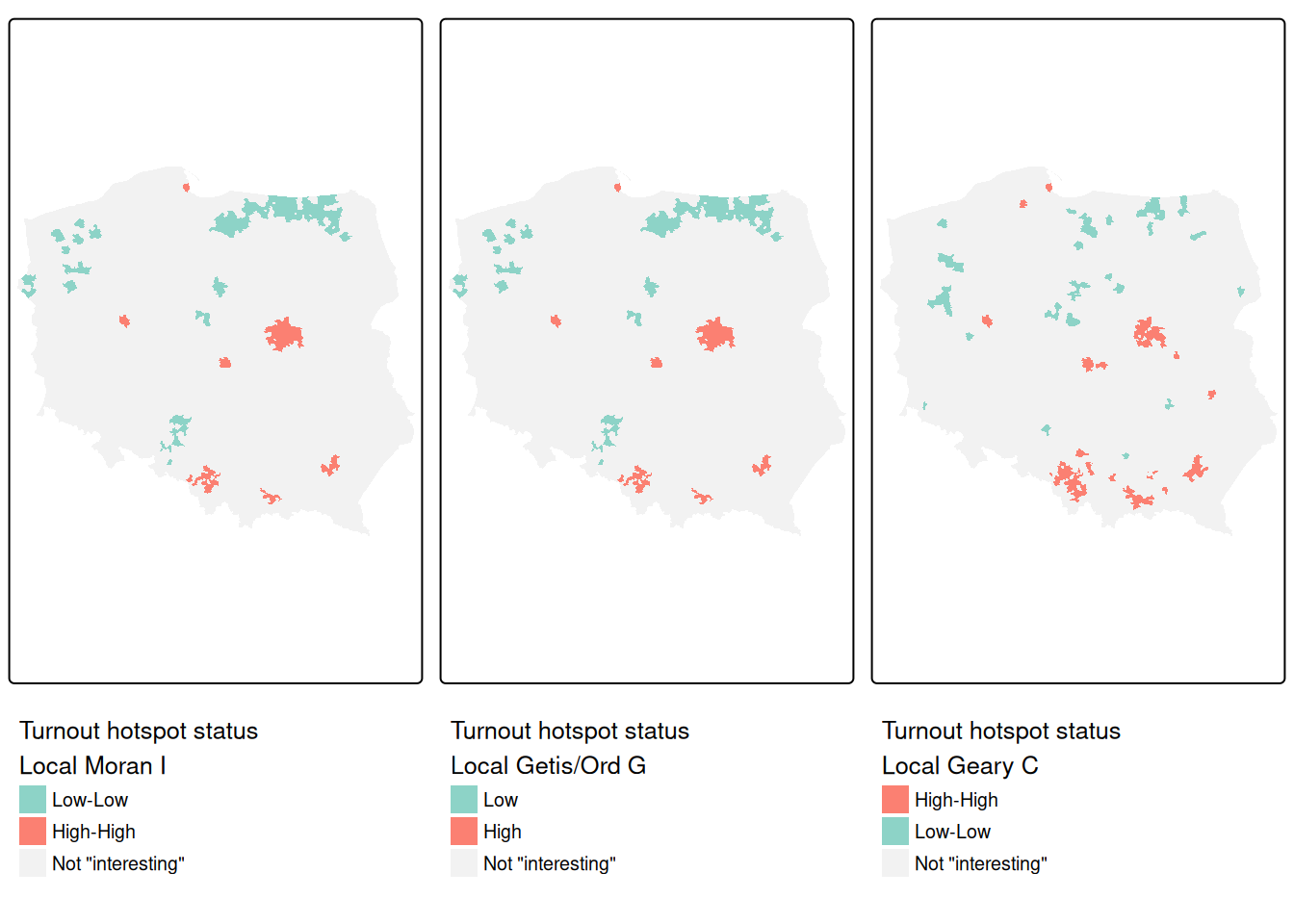Figure 15.7: FDR hotspot cluster core maps, two-sided, interesting cutoff $$\alpha = 0.005$$: left panel: local Moran's $$I_i$$; centre panel: local Getis-Ord $$G_i$$; right panel: local Geary's $$C_i$$; first-round turnout, row-standardised neighbours

Figure 15.7 shows that the cluster cores identified as interesting using $$I_i$$, $$G_i$$ and $$C_i$$ for the same variable, first-round turnout, and the same spatial weights, for rank-based permutation FDR adjusted probability values and an $$\alpha = 0.005$$ cutoff, are very similar. In most cases, the "High-High" cluster cores are urban areas, and "Low-Low" cores are sparsely populated rural areas in the North, in addition to the German national minority areas close to the southern border. The three measures use slightly different strategies for naming cluster cores: $$I_i$$ uses quadrants of the Moran scatterplot, $$G_i$$ splits into "Low" and "High" on the mean of the input variable (which is the same as the first component in the $$I_i$$ tuple), and univariate $$C_i$$ on the mean of the input variable and zero for its lag. As before, cluster categories that do not occur are dropped.

For comparison, and before moving to multivariate $$C_i$$, let us take the univariate $$C_i$$ for the second (final) round turnout. One would expect that the run-off between the two top candidates from the first-round might mobilise some voters who did not have a clear first-round preference, but that it discourages some of those with strong loyalty to a candidate eliminated after the first round:

pol_pres15 |>
st_drop_geometry() |>
subset(select = II_turnout) |>
localC_perm(lw_q_W, nsim=9999, iseed=1) -> locC_p_II
locC_p_II |> hotspot(Prname = "Pr(z != E(Ci)) Sim",
cutoff = 0.005) -> pol_pres15$hs_C_II Multivariate $$C_i$$ is taken as the sum of univariate $$C_i$$ divided by the number of variables, but permutation is fixed so that the correlation between the variables is unchanged: pol_pres15 |> st_drop_geometry() |> subset(select = c(I_turnout, II_turnout)) |> localC_perm(lw_q_W, nsim=9999, iseed=1) -> locMvC_p Let us check that the multivariate $$C_i$$ is equal to the mean of the univariate $$C_i$$: all.equal(locMvC_p, (locC_p+locC_p_II)/2, check.attributes = FALSE) #  TRUE locMvC_p |> hotspot(Prname = "Pr(z != E(Ci)) Sim", cutoff = 0.005) -> pol_pres15$hs_MvC
Code
m3 <- tm_shape(pol_pres15) +
tm_fill("hs_C",
palette = RColorBrewer::brewer.pal(4, "Set3")[c(4, 1, 3, 2)],
colorNA = "grey95", textNA = "Not \"interesting\"",
title = "First round turnout\nLocal Geary C") +
tm_layout(legend.outside=TRUE, legend.outside.position="bottom")
m4 <- tm_shape(pol_pres15) +
tm_fill("hs_C_II",
palette = RColorBrewer::brewer.pal(4, "Set3")[c(4, 1, 3, 2)],
colorNA = "grey95", textNA = "Not \"interesting\"",
title="Second round turnout\nLocal Geary C") +
tm_layout(legend.outside=TRUE, legend.outside.position="bottom")
m5 <- tm_shape(pol_pres15) +
tm_fill("hs_MvC",
palette = RColorBrewer::brewer.pal(4, "Set3")[c(4, 1)],
colorNA = "grey95", textNA = "Not \"interesting\"",
title = "Both rounds turnout\nLocal Multivariate Geary C") +
tm_layout(legend.outside=TRUE, legend.outside.position="bottom")
tmap_arrange(m3, m4, m5, nrow=1)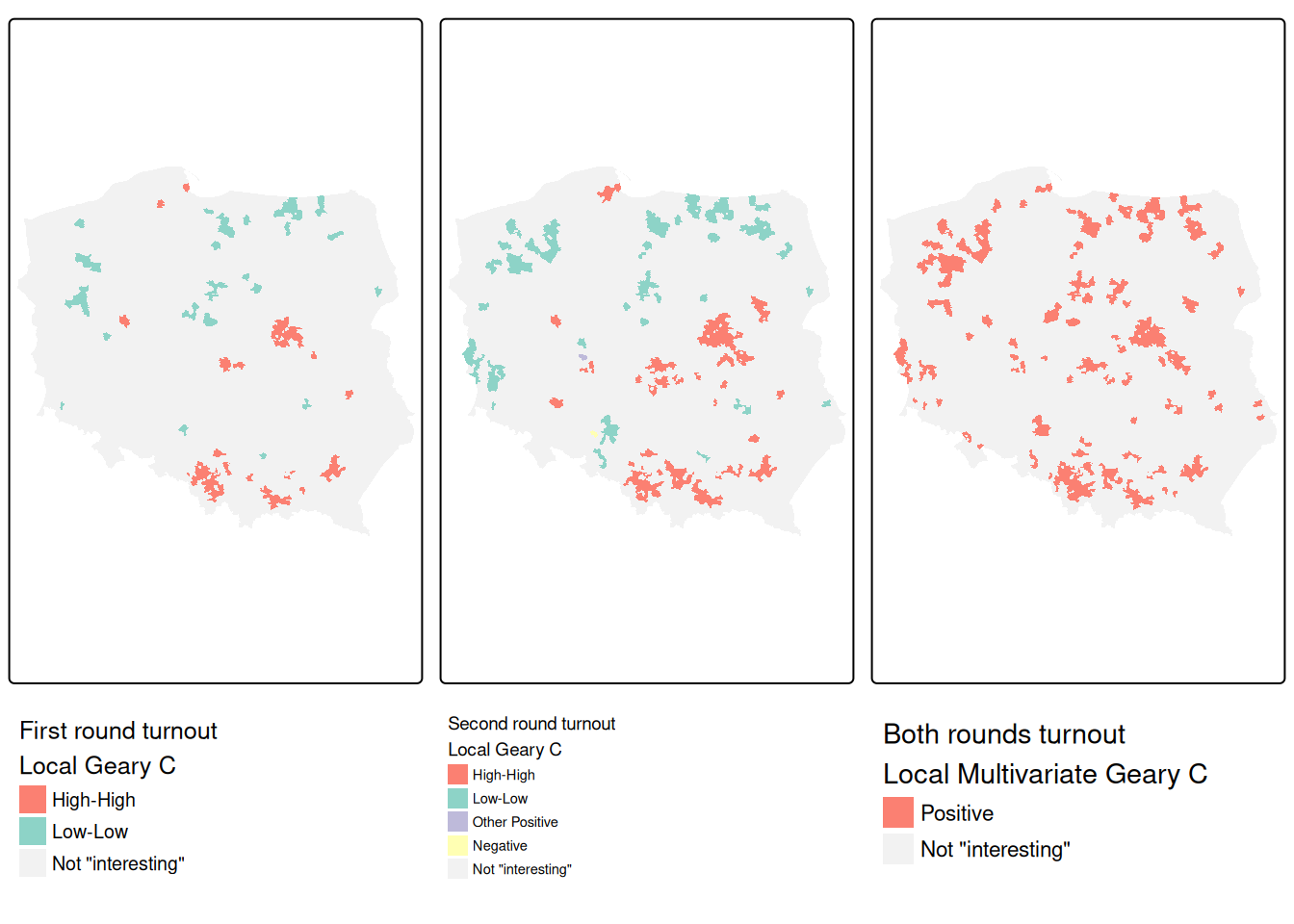Figure 15.8: FDR hotspot cluster core maps, two-sided, interesting cutoff $$\alpha = 0.005$$: left panel: local $$C_i$$, first-round turnout; centre panel: local $$C_i$$, second-round turnout; right panel: local multivariate $$C_i$$, both turnout rounds; row-standardised neighbours

Figure 15.8 indicates that the multivariate measure picks up aggregated elements of observations found interesting in the two univariate measures. We can break this down by interacting the first- and second-round univariate measures, and tabulating against the multivariate measure.

table(droplevels(interaction(addNA(pol_pres15$hs_C), addNA(pol_pres15$hs_C_II), sep=":")),
addNA(pol_pres15$hs_MvC)) # # Positive <NA> # High-High:High-High 74 0 # NA:High-High 55 16 # Low-Low:Low-Low 24 0 # NA:Low-Low 53 11 # NA:Other Positive 1 0 # High-High:NA 13 4 # Low-Low:NA 8 3 # NA:NA 73 2160 For these permutation outcomes, 47 observations in the multivariate case are found interesting where neither of the univariate $$C_i$$ were found interesting (FDR, cutoff $$0.005$$). Almost all of the observations found interesting in both first and second round, are also interesting in the multivariate case, but outcomes are more mixed when observations were only found interesting in one of the two rounds. ### The rgeoda package Geoda has been wrapped for R as rgeoda , and provides very similar functionalities for the exploration of spatial autocorrelation in areal data as matching parts of spdep. The active objects are kept as pointers to a compiled code workspace; using compiled code for all operations (as in Geoda itself) makes rgeoda perform fast, but makes it less flexible when modifications or enhancements are desired. library(rgeoda) Geoda_w <- queen_weights(pol_pres15) summary(Geoda_w) # name value # 1 number of observations: 2495 # 2 is symmetric: TRUE # 3 sparsity: 0.00228786229774178 # 4 # min neighbors: 1 # 5 # max neighbors: 13 # 6 # mean neighbors: 5.70821643286573 # 7 # median neighbors: 6 # 8 has isolates: FALSE For comparison, let us take the multivariate $$C_i$$ measure of turnout in the two rounds of the 2015 Polish presidential election as above: lisa <- local_multigeary(Geoda_w, pol_pres15[c("I_turnout", "II_turnout")], cpu_threads = max(detectCores() - 1, 1), permutations = 99999, seed = 1) The contiguity neighbours are the same as those found by poly2nb: all.equal(card(nb_q), lisa_num_nbrs(lisa), check.attributes = FALSE) #  TRUE as are the multivariate $$C_i$$ values the same as those found above: all.equal(lisa_values(lisa), c(locMvC_p), check.attributes = FALSE) #  TRUE One difference is that the range of the folded two-sided rank-based permutation probability values used by rgeoda is $$[0, 0.5]$$, also reported in spdep: apply(attr(locMvC_p, "pseudo-p")[,c("Pr(z != E(Ci)) Sim", "Pr(folded) Sim")], 2, range) # Pr(z != E(Ci)) Sim Pr(folded) Sim # [1,] 0.0002 0.0001 # [2,] 0.9988 0.4994 This means that the cutoff corresponding to $$0.005$$ over $$[0, 1]$$ is $$0.0025$$ over $$[0, 0.5]$$: locMvC_p |> hotspot(Prname = "Pr(folded) Sim", cutoff = 0.0025) -> pol_pres15$hs_MvCa

So although local_multigeary used the default cutoff of $$0.05$$ in setting cluster core classes, we can sharpen the cutoff and apply the FDR adjustment on output components of the lisa object in the compiled code workspace:

mvc <- factor(lisa_clusters(lisa), levels=0:2,
labels = lisa_labels(lisa)[1:3])
is.na(mvc) <- p.adjust(lisa_pvalues(lisa), "fdr") >= 0.0025
pol_pres15$geoda_mvc <- droplevels(mvc) About 80 more observations are found interesting in the rgeoda permutation, and further analysis of implementation details is still in progress: addmargins(table(spdep = addNA(pol_pres15$hs_MvCa),
#           rgeoda
# spdep      Positive <NA>  Sum
#   Positive      292    9  301
#   <NA>           35 2159 2194
#   Sum           327 2168 2495
Code
m5 <- tm_shape(pol_pres15) +
tm_fill("hs_MvCa",
palette = RColorBrewer::brewer.pal(4, "Set3")[c(4, 1)],
colorNA = "grey95", textNA = "Not \"interesting\"",
title = "Both rounds turnout spdep\nLocal Multivariate Geary C")
m6 <- tm_shape(pol_pres15) +
tm_fill("geoda_mvc",
palette = RColorBrewer::brewer.pal(4, "Set3")[c(4, 1)],
colorNA = "grey95", textNA = "Not \"interesting\"",
title="Both rounds turnout rgeoda\nLocal Multivariate Geary C")
tmap_arrange(m5, m6, nrow=1)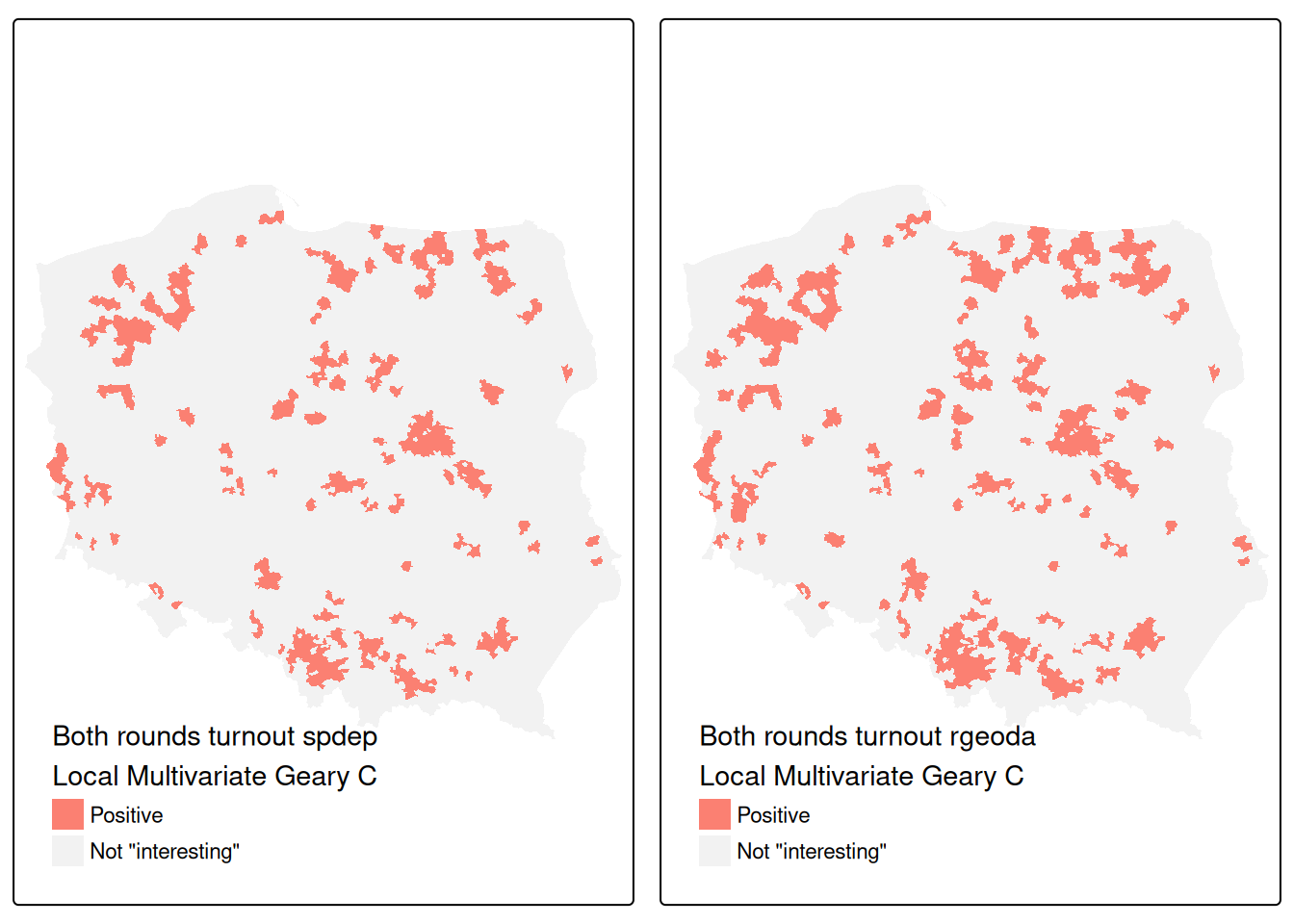Figure 15.9: FDR local multivariate $$C_i$$ hotspot cluster core maps, two-sided, interesting cutoff $$\alpha = 0.0025$$ over $$[0, 0.5]$$: left panel: spdep, both turnout rounds; right panel: rgeoda, both turnout rounds; row-standardised neighbours

Figure 15.9 shows that while almost all of the 242 observations found interesting in the spdep implementation were also interesting for rgeoda, the latter found a further 86 interesting. Of course, permutation outcomes are bound to vary, but it remains to establish whether either or both implementations require revision.

## 15.4 Exercises

1. Why are join-count measures on a chessboard so different between rook and queen neighbours?
2. Please repeat the simulation shown in Section 15.1 using the chessboard polygons and the row-standardised queen contiguity neighbours. Why is it important to understand that spatial autocorrelation usually signals (unavoidable) misspecification in our data?
3. Why is false discovery rate adjustment recommended for local measures of spatial autocorrelation?
4. Compare the local Moran’s $$I_i$$ standard deviate values for the simulated data from exercise 2 (above) for the analytical conditional approach, and saddlepoint approximation. Consider the advantages and disadvantages of the saddlepoint approximation approach.